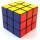# Cube volume

The cube has a surface of 384 cm2. Calculate its volume.

Result

V =  512 cm3

#### Solution:Leave us a comment of example and its solution (i.e. if it is still somewhat unclear...):

Showing 0 comments:Be the first to comment!#### To solve this example are needed these knowledge from mathematics:

Tip: Our volume units converter will help you with converion of volume units.

## Next similar examples:

1. Volume from surface areaWhat is the volume of the cube whose surface area is 96 cm2?
2. Cube 1-2-3Calculate the volume and surface area of the cube ABCDEFGH if: a) /AB/ = 4 cm b) perimeter of wall ABCD is 22 cm c) the sum of the lengths of all edges of the cube is 30 cm.
3. CuboidFind the cuboid that has the same surface area as the volume.
4. Cube wallsFind the volume and the surface area of the cube if the area of one of its walls is 40 cm2.
5. Reduce of the volumeCalculate how many % reduce the volume of the cube is we reduced length of each edge by 10%.
6. Cube 7Calculate the volume of a cube, whose sum of the lengths of all edges is 276 cm.
7. Water levelThe glass container has a cuboid shape with dimensions of the bottom 24 cm and 12 cm. The height of water is 26 cm. Calculate the volume of the body, which are sunk into the water if the water level rise by 3 cm.
8. Cube cornersThe wooden cube with edge 64 cm was cut in 3 corners of cube with edge 4 cm. How many cubes of edge 4 cm can be even cut?
9. RainfallThe annual average rainfall in India was in Cherrapunji in the year 1981 26 461 mm. How many hectoliters of water fell on 1 m2? Would fit this amount of water into a cube of three meters?
10. CubesCube, which consists of 27 small cubes with edge 4 dm has volume:
11. Cube cornersFrom cube of edge 14 cm cut off all vertices so that each cutting plane intersects the edges 1 cm from the nearest vertice. How many edges will have this body?
12. Cube surface areaThe surface of the cube was originally 216 centimeters square. The surface of the cube has shrunk from 216 to 54 centimeters sq. Calculate how much percent the edge of the cube has decreased.
13. Cone area and sideCalculate the surface area and volume of a rotating cone with a height of 1.25 dm and 17,8dm side.
14. Triangular prismCalculate the surface area and volume of a triangular prism, base right triangle if a = 3 cm, b = 4 cm, c = 5 cm and height of prism h=12 cm.
15. Truncated coneA truncated cone has a bases radiuses 40 cm and 10 cm and a height of 25 cm. Calculate its surface area and volume.
16. Cylinder surface areaVolume of a cylinder whose height is equal to the radius of the base is 678.5 dm3. Calculate its surface area.
17. Fit ballWhat is the size of the surface of Gymball (FIT - ball) with a diameter of 65 cm?